Electron. J. Diff. Equ., Vol. 2011 (2011), No. 19, pp. 1-21.

### Quadratic forms as Lyapunov functions in the study of stability of solutions to difference equations Alexander O. Ignatyev, Oleksiy Ignatyev

Abstract:
A system of linear autonomous difference equations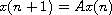is considered, where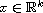,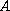is a real nonsingular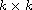matrix. In this paper it has been proved that if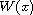is any quadratic form andis any positive integer, then there exists a unique quadratic form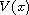such that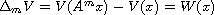holds if and only if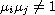(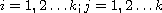) where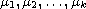are the roots of the equation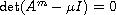.
A number of theorems on the stability of difference systems have also been proved. Applying these theorems, the stability problem of the zero solution of the nonlinear system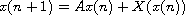has been solved in the critical case when one eigenvalue of a matrixis equal to minus one, and others lie inside the unit disk of the complex plane.

Submitted February 1, 2010. Published February 3, 2011.
Math Subject Classifications: 39A11, 34K20.
Key Words: Difference equations; Lyapunov function.

Show me the PDF file (353 KB), TEX file, and other files for this article.Alexander O. Ignatyev Institute for Applied Mathematics and Mechanics R. Luxemburg Street,74, Donetsk-83114, Ukraine email: aoignat@mail.ru, ignat@iamm.ac.donetsk.ua Oleksiy Ignatyev Department of Statistics and Probability Michigan State University A408 Wells Hall, East Lansing, MI 48824-1027, USA email: ignatyev@stt.msu.edu, aignatye@math.kent.edu